# Physical Quantities Units and Measurements Quiz 1

#### Quiz Description

Here in this quiz, we shall be having a broader view of the various concepts under Physical quantities, units, measurements, and dimensions. You shall be tested on the identification of measurement parameters, calculation of error, and your understanding of SI units among others. It is going to be an insightful activity taking the quiz.

A physical quantity could be defined as a body or material property that can be quantified and measured. After quantification and measurement, the final results need to be expressed with respect to some standards, and this is when units come in. Units are standards for measurement. Some examples of units are: kilogram (kg), meter(m), Ampere(A), etc. Physical quantities can also be further divided into vectors (physical quantities with magnitude and direction) and scalar quantities (physical quantities with magnitude but no direction).

You might have been having doubts concerning this topic. Here is your chance to clear your mind by solving the quiz. We wish you the best as you practice alongside other exciting physics quizzes

The diagram shows arrows representing two vector quantities.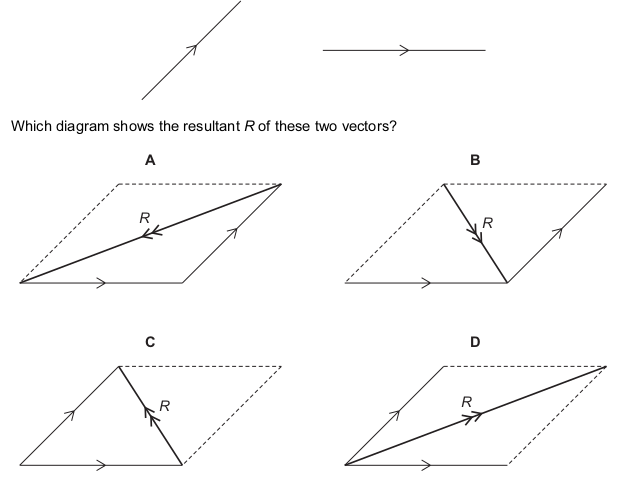Correct
• A
• B
• C
• D

Which set of quantities are all vectors?

Correct
• acceleration, displacement, velocity
• chemical energy, mass, power
• extension, force, gravitational potential energy
• weight, kinetic energy, work

A student determines the circumference of a golf ball.

Which instrument gives a reading that is the circumference of the golf ball?

Correct
• calipers
• micrometer
• rule
• tape

Which quantity is a vector?

Correct
• energy
• force
• speed
• time

Is mass a scalar or a vector, and is acceleration a scalar or a vector?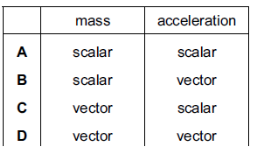Correct
• A
• B
• C
• D

The diameter and the length of a thin wire, approximately 50 cm in length, are measured as precisely as possible.

What are the best instruments to use?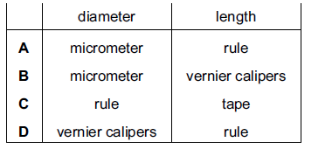Correct
• A
• B
• C
• D

Newton’s third law involves two quantities which are equal in size and opposite in direction.

What is the unit for these two quantities?

Correct
• J
• m / s 2
• N
• W

Which quantity is a scalar?

Correct
• acceleration
• force
• temperature
• velocity

During an experiment to find the density of a stone, the stone is lowered into a measuring cylinder partly filled with water.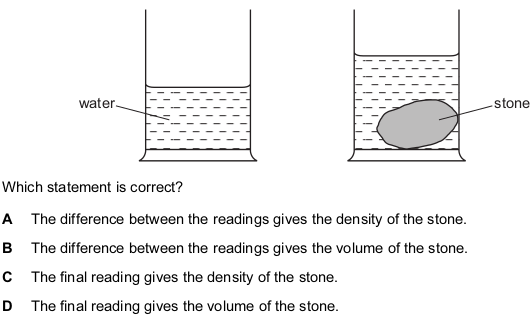Correct
• A
• B
• C
• D

The diagram shows a micrometer scale.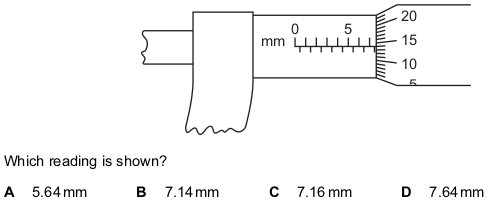Correct
• A
• B
• C
• D

Which is a scalar quantity?

Correct
• mass
• force
• velocity
• weight

A workman measures, as accurately as possible, the length and internal diameter of a straight copper pipe.

The length is approximately 600 cm and the internal diameter is approximately 2 cm.

What is the best combination of instruments for the workman to use?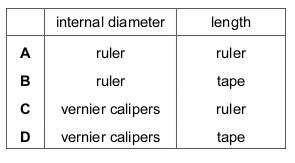Correct
• A
• B
• C
• D

The diagram shows a stopwatch.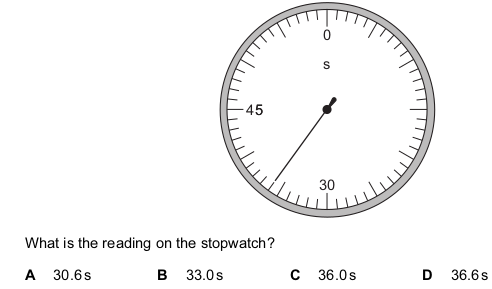Correct
• A
• B
• C
• D

Each row contains a vector and a scalar.

In which row is the size of the vector equal to the size of the scalar?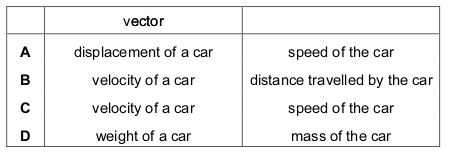Correct
• A
• B
• C
• D

What is the size of the resultant of the two forces shown in the diagram?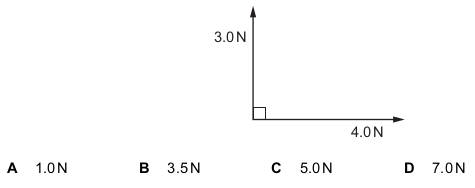Correct
• A
• B
• C
• D

Forces of 25 N and 40 N act on an object in the directions shown.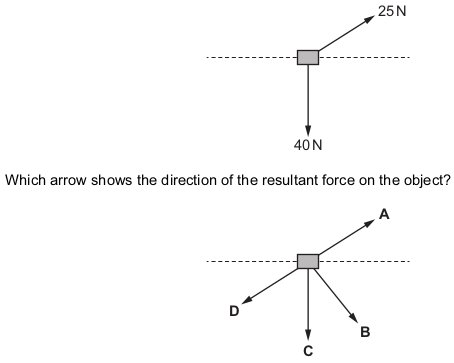Correct
• A
• B
• C
• D

Which device can be used to measure the thickness of a single sheet of paper?

Correct
• a meter rule
• a micrometer
• a plastic ruler
• a measuring tape

In a test, four students linked the quantities on the left with their units on the right.

Which student matched them all correctly?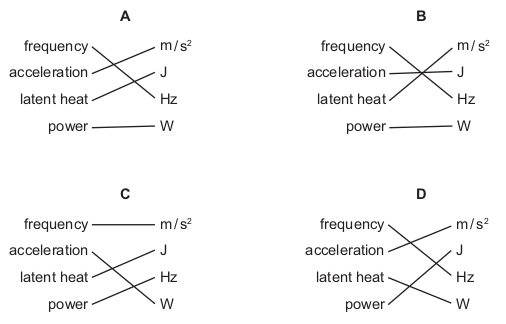Correct
• A
• B
• C
• D

Forces of 3 N and 4 N act as shown in the diagram.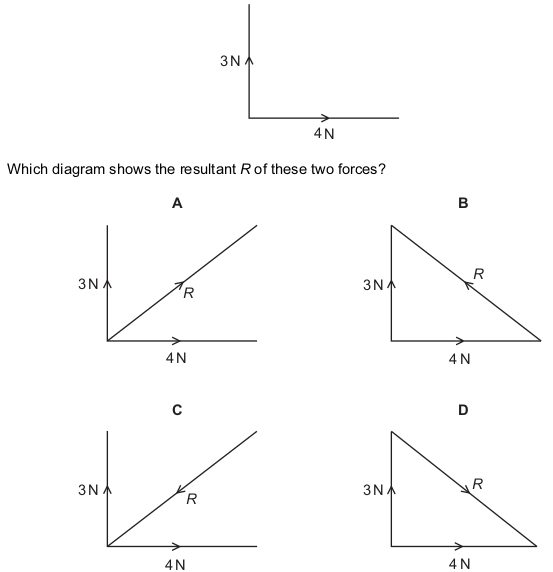Correct
• A
• B
• C
• D

A micrometer is used to measure the diameter of a uniform wire.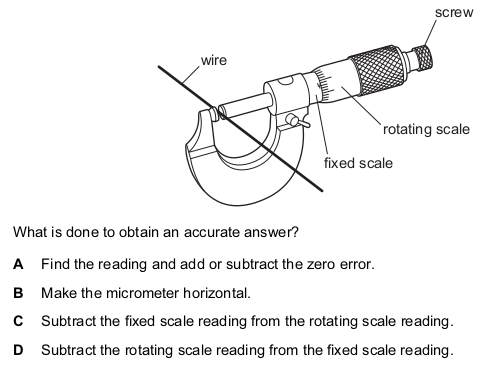Correct
• A
• B
• C
• D

Before marking the finishing line on a running track, a groundsman measures out its 100 m length.

Which instrument is the most appropriate for this purpose?

Correct
• measuring tape
• meter rule
• 30 cm ruler
• micrometer